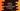# 4 ways to convert a datetime object to epoch in Python## How to convert a datetime object to epoch in Python:

There are different ways to convert a python datetime object to epoch in Python. Epoch time is also known as unix time or posix time or number of seconds since epoch. It is the number of seconds since 1st January, 1970 00:00:00 UTC.

datetime module is used to work with different date-time related operations in Python.

We can convert a datetime object to epoch in different ways in Python. In this post, I will show you different ways to do that with examples.

### Constructor of datetime:

The constructor of datetime is:

``datetime(year, month, day[, hour[, minute[, second[, microsecond[,tzinfo]]]]])``

Here, year, month and day are required arguments. Other arguments are optional. We can pass None to tzinfo or we can pass an instance of tzinfo class. The arguments can be integers.

### Method 1: By using strftime:

strftime method is used to convert a datetime object to a string. It takes a format type and returns the converted string.

We can pass %s to strftime to get the epoch time.

But, this is not recommended and it will fail on Windows system. This is not a supported format type. It works on unix system because internally it calls system’s strftime method and that uses the system’s timezone.

``````from datetime import datetime

d = datetime(2021,5,2,0,0)

print(d.strftime('%s'))``````

It will print 1619893800.

### Method 2: By using timestamp():

The timestamp() method is introduced in Python 3.3. If your project supports Python 3.3, you can directly use this method.

``````from datetime import datetime

d = datetime(2021,5,2,0,0)

print(d.timestamp())``````

Datetime object uses system time. If you want to get it in UTC timezone, you need to convert the datetime object’s timezone first.

``````from datetime import datetime
from datetime import timezone

d = datetime(2021,5,2,0,0)

print(d.replace(tzinfo=timezone.utc).timestamp())``````

### Method 3: By using total_seconds():

timestamp() method internally uses total_seconds method to calculate the value. If you are not using Python 3.3, you can use this way to convert a datetime object to epoch.

So, the idea is to subtract 1st January, 1970, 00:00:00 UTC datetime from the given datetime and by using total_seconds() method with the result.

``````from datetime import datetime

d = datetime(2021,5,2,0,0)

print((d - datetime(1970,1,1)).total_seconds())``````

### Method 4: By using calendar module:

There is a method called timegm that is defined in the calendar module. This method takes a time tuple and returns a unix timestamp value from that tuple. We can also use timetuple method of datetime and pass that value to timegm to get the unix time.

For example:

``````from datetime import datetime
import calendar

d = datetime(2021,5,2,0,0)

print(calendar.timegm(d.timetuple()))``````

### Examples of all methods:

Let’s try all of these examples in one program:

``````from datetime import datetime
from datetime import timezone
import calendar

d = datetime(2021,5,2,0,0)

print(d.strftime('%s'))
print(d.timestamp())
print((d - datetime(1970,1,1)).total_seconds())
print(calendar.timegm(d.timetuple()))``````